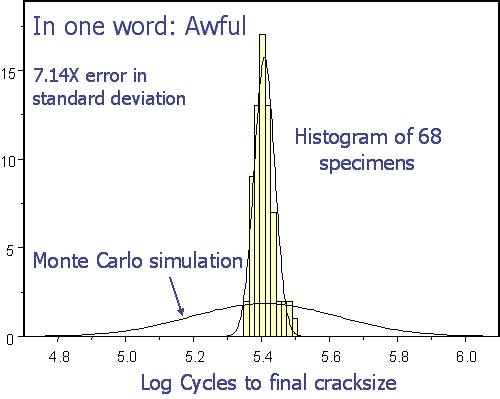## Probabilistics 4

### “Probabilistics” 4

There is more to Monte Carlo simulation than replacing constants with probability densities.The conventional MC simulation of 1000 samples, with independent model parameters, $$C$$ and $$n$$, did an acceptable job predicting the mean lifetime, after the log transform.

The simulated standard deviation was another matter: The conventional MC simulation of 1000 samples, with independent model parameters, $$C$$ and $$n$$, produced a standard deviation 700% too large!

This absurd simulation result has been observed by every engineer who has performed similar MC simulations, since it doesn’t require any statistics to detect an answer that is wrong by a factor approaching an order of magnitude in standard deviation. Sadly the most common palliatives proposed as remedies do not perform much better.

(Continued …)